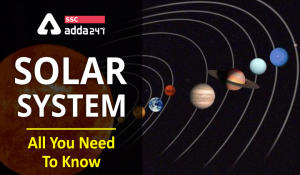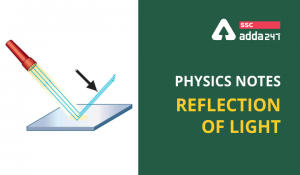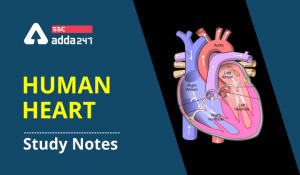Latest SSC jobs   »   Electric Current Notes : Overview and...

# Electric Current Notes : Overview and Important Points 2021

General Science is one of the most important sections with respect to all Government Job Exams whether it is SSC CGL or RRB NTPC CBT 2, which can help a candidate in scoring maximum marks in a competitive exam in the least amount of time. You don’t need to perform the complex calculations to settle for the correct option so, it’s best to be prepared with the facts and figures in advance so as to score to the maximum in this section. Here we are providing important notes related to Physics, detailed notes on definitions, concepts, laws, formulae, rules, and properties, important from the exam point of view.

# Electric Current

Electric current is expressed by the amount of charge flowing through a particular area in unit time. It is the rate of flow of electric charges. If a net charge Q, flows across any cross-section of a conductor in time t, then the current I, through the cross-section is-

I = Q/t

The SI unit of electric charge is the coulomb (C), which is equivalent to the charge contained in nearly: 6 ✖ 10¹⁸ electrons

The electric current is expressed by a unit called ampere (A). One ampere is constituted by the flow of one coulomb of charge per second, that is, 1A = 1C/1s.

EXAMPLE: A current of 0.5 A is drawn by a filament of an electric bulb for 10 minutes. Find the amount of electric charge that flows through the circuit.
Solution:
We are given, I = 0.5 A; t = 10 min = 600 s.
As Q = It
= 0.5 A × 600 s
= 300 C

ELECTRIC POTENTIAL AND POTENTIAL DIFFERENCE

The electric potential difference between two points in an electric circuit carrying some current is the work done to move a unit charge from one point to the other

Potential difference (V) between two points = Work done (W)/Charge (Q)
V = W/Q

The SI unit of electric potential difference is volt (V), named after Alessandro Volta (1745–1827), an Italian physicist. One volt is the potential difference between two points in a current-carrying conductor when 1 joule of work is done to move a charge of 1 coulomb from one point to the other.

The potential difference is measured by means of an instrument called the voltmeter. The voltmeter is always connected in parallel across the points between which the potential difference is to be measured.

Study Notes On Tughlaq Dynasty: Rulers, Dynasty and a Complete Overview

National Emergency Definition, Introduction, Types: Article 352 and FAQs

ELECTRIC CURRENTS IN CONDUCTORS

In other materials, notably metals, some of the electrons are practically free to move within the bulk material. These materials, generally called conductors, develop electric currents in them when an electric field is applied.

In solid conductors:

• When no electric field is present- The number of electrons traveling in any direction will be equal to the number of electrons traveling in the opposite direction. So, there will be no net electric current.
• If an electric field is applied- An electric field will be created and is directed from the positive towards the negative charge. They will thus move to neutralize the charges. The electrons, as long as they are moving, will constitute an electric current.

Study Notes On Gupta Dynasty: Rulers, Overview, Important Points and FAQs

OHM’S LAW

Imagine a conductor through which current I is flowing and let V be the potential difference between the ends of the conductor.Ohm’s law states that:

V ∝ I
or, V = R I;

where the constant of proportionality R is called the resistance of the conductor. The SI units of resistance is ohm, and is denoted by the symbol Ω.

The resistance of the conductor depends-

• on its length,
• on its area of cross-section, and
• on the nature of its material.

LIMITATIONS OF OHM’S LAW

• V ceases to be proportional to I
• The relation between V and I depends on the sign of V. In other words, if I is the current for a certain V, then reversing the direction of V keeping its magnitude fixed, does not produce a current of the same magnitude as I in the opposite direction
• The relation between V and I is not unique, i.e., there is more than one value of V for the same current I A material exhibiting such behavior is GaAs.

RESISTIVITY

The resistance of a uniform metallic conductor is directly proportional to its length (l) and inversely proportional to the area of cross-section (A). That is,

R ∝ l and
R ∝ 1/A

Combining both, we get

R ∝ l/A
or, R = ρl/A

where ρ (rho) is a constant of proportionality and is called the electrical resistivity of the material of the conductor. The SI unit of resistivity is Ω m. It is a characteristic property of the material.

List of Governor-General of India: Overview, Important Points and FAQs 2021

RESISTIVITY OF VARIOUS MATERIALS

• The resistivity of a material is found to be dependent on the temperature.
• The resistivity of an alloy is generally higher than that of its constituent metals. Alloys do not oxidize (burn) readily at high temperatures. For this reason, they are commonly used in electrical heating devices, like electric iron, toasters, etc. Tungsten is used almost exclusively for filaments of electric bulbs, whereas copper and aluminum are generally used for electrical transmission lines.
• Some materials like Nichrome (which is an alloy of nickel, iron, and chromium) exhibit a very weak dependence of resistivity with temperature.
• Unlike metals, the resistivities of semiconductors decrease with increasing temperatures.

TWO MAJOR TYPES OF RESISTORS:

• Wire bound resistors: They are made by winding the wires of an alloy, viz., manganin, constantan, nichrome or similar ones. The choice of these materials is dictated mostly by the fact that their resistivities are relatively insensitive to temperature. These resistances are typically in the range of a fraction of an ohm to a few hundred ohms.
• Carbon Resistors: Resistors in the higher range are made mostly from carbon. Carbon resistors are compact, inexpensive and thus find extensive use in electronic circuits. Carbon resistors are small in size and hence their values are given using a colour code.

RELATION OF CURRENT AND DRIFT VELOCITY

• When an electric field is applied, inside the conductor due to electric force the path of an electron, in general, becomes curved (parabolic) instead of straight lines, and electrons drift opposite to the field figure (B).
• Due to this drift, the random motion of electrons gets modified and there is a net transfer of electrons across a cross-section resulting in current.
• Drift velocity is the average uniform velocity acquired by free electrons inside a metal by the application of an electric field which is responsible for current through it.

ELECTRICAL ENERGY AND POWER

The rate at which electric energy is dissipated or consumed in an electric circuit is termed as electric power. The power P is given by

P = VI
Or P = I²R = V²/R

The SI unit of electric power is the watt (W). It is the power consumed by a device that carries 1 A of current when operated at a potential difference of 1 V. Thus,

1 W = 1 volt × 1 ampere = 1 V A

The commercial unit of electric energy is kilowatt-hour (kW h), commonly known as ‘unit’

CELLS IN SERIES AND IN PARALLEL

Cell: A cell is a device that generates electricity by using chemical energy. A cell has two electrodes, called the positive (P) and the negative (N), immersed in an electrolytic solution where the electrodes exchange charges with the electrolyte.

Note:-

• Current flows from cathode to anode through an external circuit.
• Current flows from anode to cathode through the electrolyte.

EMF (Electromotive force): It is defined as the potential difference between electrodes when there is no current in the cell.Emf of the cell initiates the flow of current in the cell.

Internal Resistance: It is the resistance offered by the electrolyte and electrodes when the current flows. It is denoted by ‘r’

Cells in Series

• When multiple cells are arranged in such a way that the positive terminal of one cell is connected to the negative terminal of the other cell and so on, it is known to be in series combination.
• For two cells of emf’s E1 and E2 connected in series with r1, r2 as their internal resistances, the formula is given as:
E equivalent = E1 + E2
r equivalent = r1 + r2

The rule for cells arranged in series combination:

• The equivalent emf of a series combination of n cells is just the sum of their individual emf’s, and
• The equivalent internal resistance of a series combination of n cells is just the sum of their internal resistances.

Cells in Parallel

• When cells are arranged in such a way that the positive terminals of all the cells are connected together and all the negative terminals are connected together, it is known to be in parallel combination.
• For two cells of emf’s E1 and E2 connected in parallel with r1, r2 as their internal resistances, the formula is given as:
1/r equivalent = 1/r₁ + 1/r₂
E equivalent/ r eq = E₁/r₁ + E₂/r₂

Important Note:-

• Cells are arranged in series to increase the voltage.
• Cells are increased in parallel to increase the current.

POTENTIOMETER

• It is basically a long piece of uniform wire, sometimes a few meters in length across which a standard cell is connected.
• A current I flows through the wire which can be varied by a variable resistance (rheostat, R) in the circuit. Since the wire is uniform, the potential difference between A and any point at a distance l from A is
E(l) = ⌽l
where ⌽ is the potential drop per unit length.An application of the potentiometer is to compare the emf of two cells of emf ε1 and ε2 which is given by the equation:
E1/E2 = l1/l2
• The potentiometer has the advantage that it draws no current from the voltage source being measured.
• Potentiometer is also used to measure the internal resistance of a cell.

Conductor:

• A substance that allows the passage of electric charges through it easily is called a conductor. A conductor offers very low resistance to the flow of current. For example copper, silver, aluminum etc.

Insulator

• A substance that has infinitely high resistance does not allow electric current to flow through it. It is called an insulator. For example rubber, glass, plastic, Ebonite etc.

Coulomb’s Law:

• The mutual electrostatic force between two point charges q₁ and q₂ is proportional to the product q₁ q₂ and inversely proportional to the square of the distance r₂₁ separating them.

#### Congratulations!

General Awareness & Science Capsule PDF

Incorrect details? Fill the form again here

General Awareness & Science Capsule PDF

Thank You, Your details have been submitted we will get back to you.
•Our Solar System, Formation, Planets, Fa...
•Study Notes On "BLOOD" For SSC & Rai...
•Reflection of Light: Definition, Types, ...
•Physics notes For SSC & Railway Exam...
•Study notes on “Human Heart” For Gov...
•हमारा सौरमंडल: �...